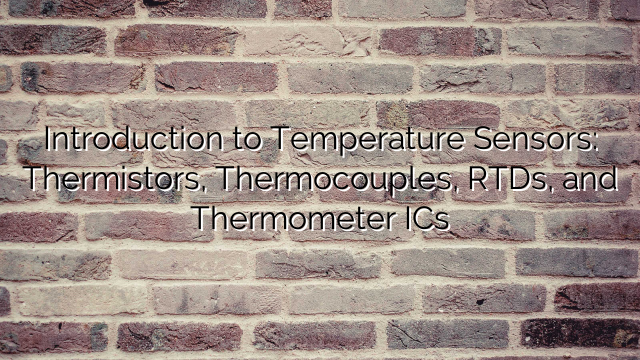Introduction to Temperature Sensors: Thermistors, Thermocouples, RTDs, and Thermometer ICs

As the name implies, the thermistor (i.e., thermal resistor) is a temperature-sensing device whose resistance is a function of its temperature.

Thermistors are available in two types: PTC (positive temperature coefficient) and NTC (negative temperature coefficient). The resistance of a PTC thermistor increases as the temperature increases. In contrast, the resistance of an NTC thermistor decreases as temperature increases, and this type seems to be the most commonly used thermistor. See Figure 1 below.

For More Details:
Event promo video